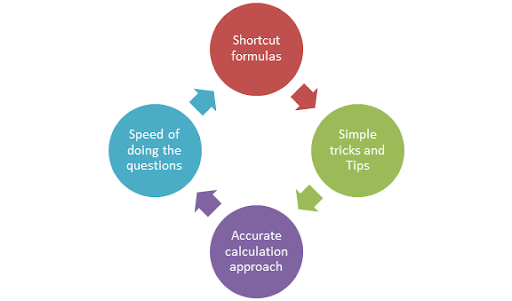## Posts

### NDA, AIR FORCE, NAVY, ALL DEFENCE ACADEMY SOLUTIONSThe math’s curriculum of  AFCAT  is basically 8 topics of quantitative aptitude. These topics with the  highest weightage to lowest weightage of questions in AFCAT Numerical Ability Section  which includes around  18  questions are; Speed, Distance and Time Work and Time Percentage Average Simple and Compound interest Ratio & Proportion Profit & Loss Decimal Fraction/ Simplification In AFCAT exam mathematics is the subject from where you can score 100%. Though questions in Numerical Ability Section are based on simple 10 th  level arithmetic, what one needs to be good with are  the Important Formulas, Simple tricks and tips, Accurate calculation-based approach and finally Improves one’s speed of doing the questions  which are interconnected and dent as a cycle of shortcut bunch of Best confidence to have in one’s to appear for any question in  Numerical Ability Section  of your AFCAT 2020 exam paper. This article gives you a clear idea